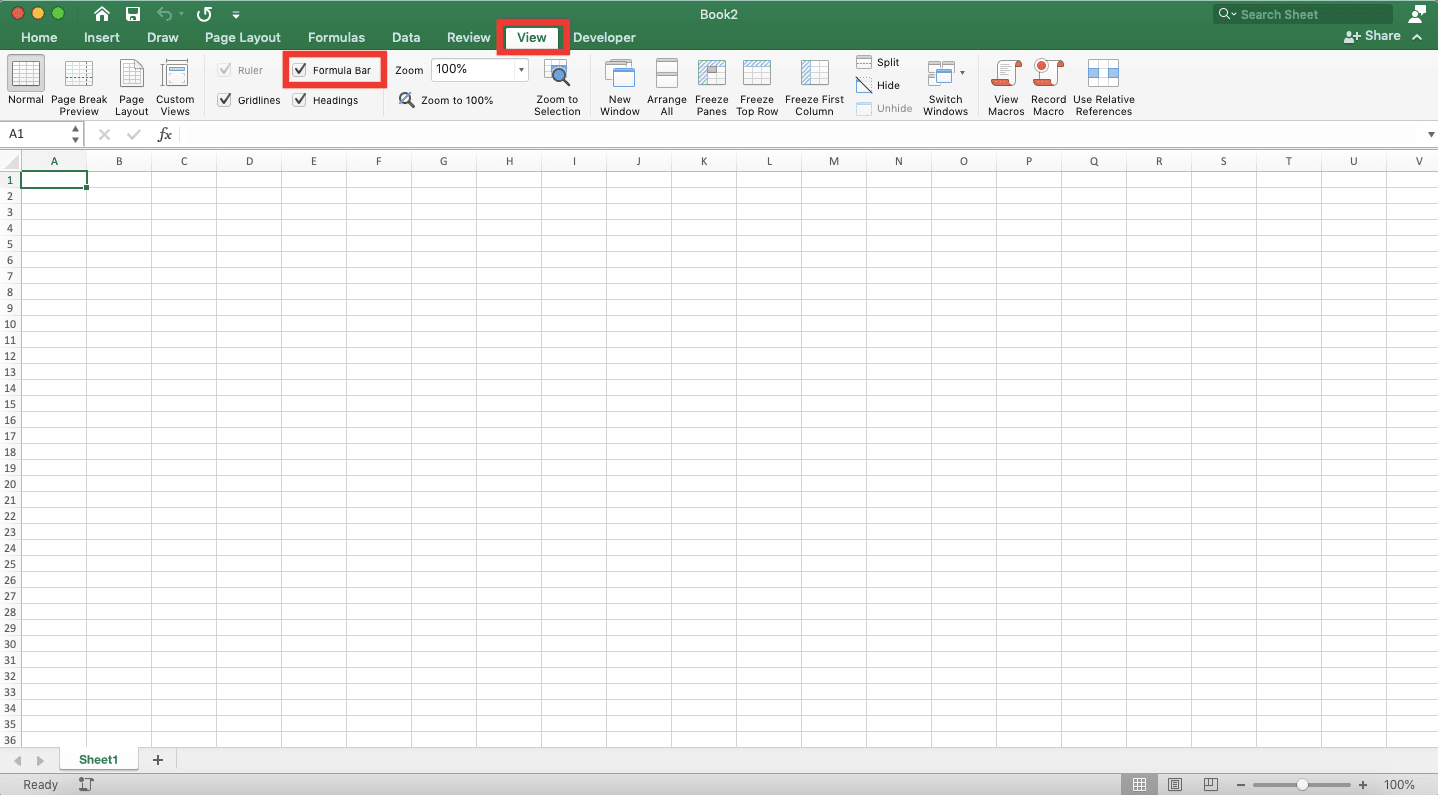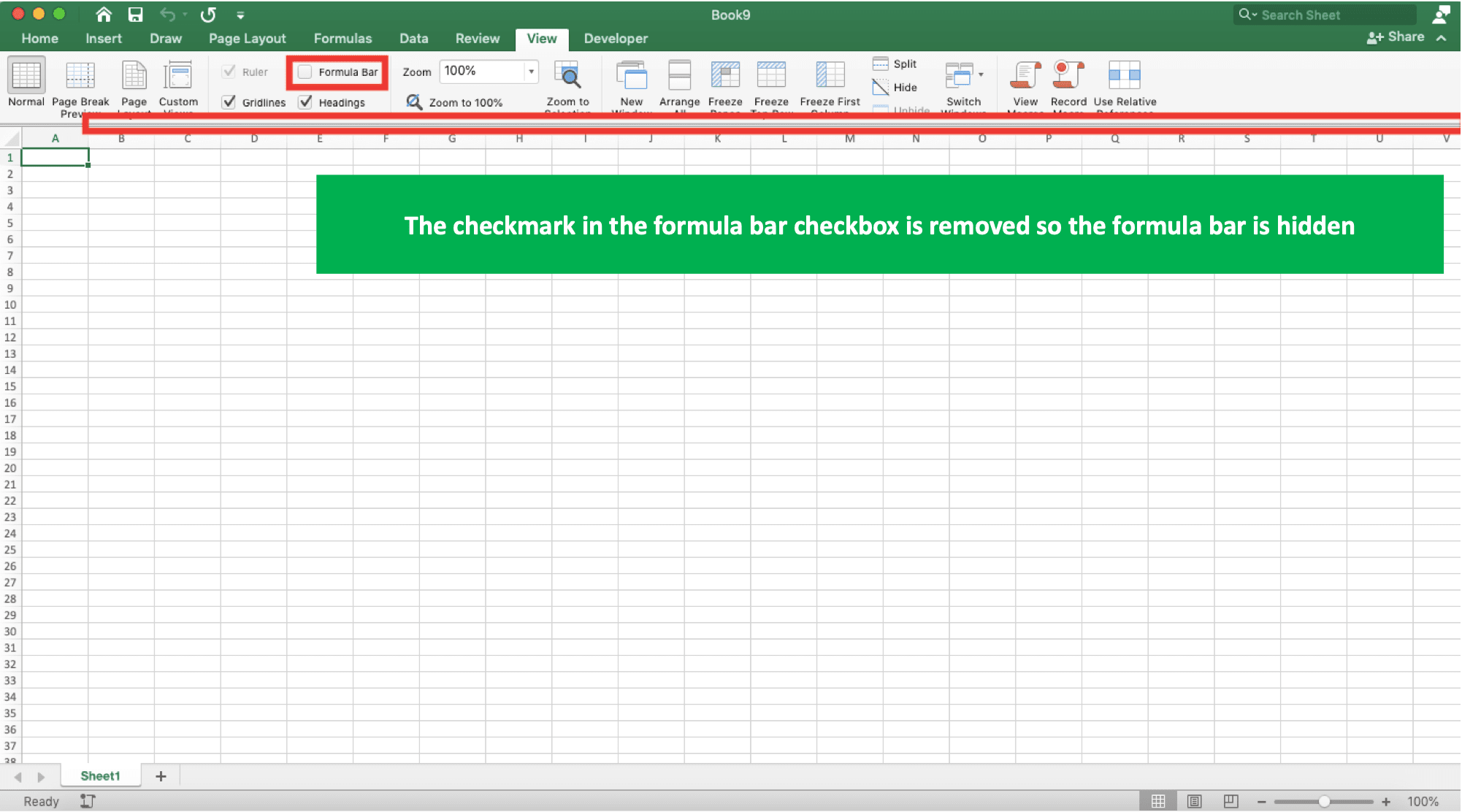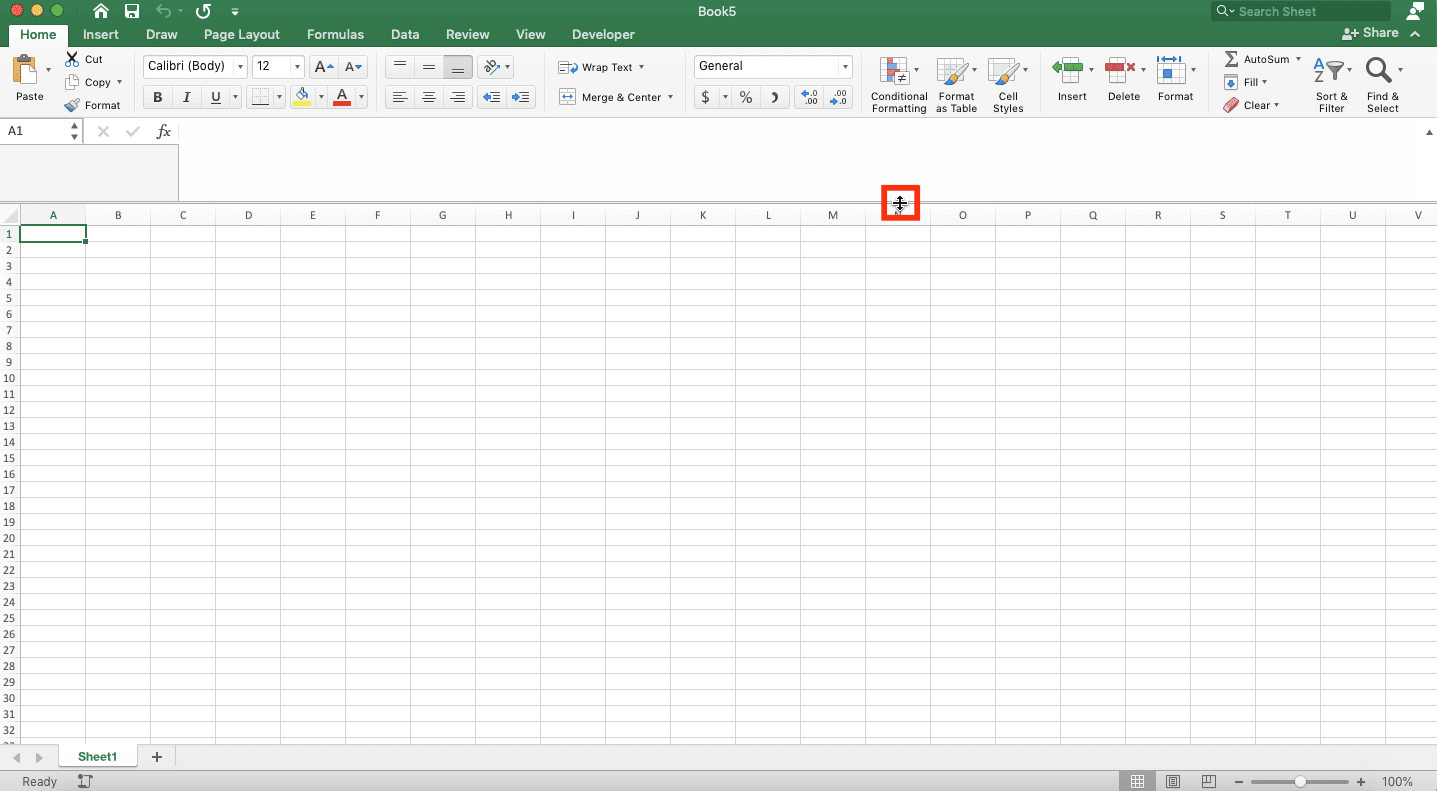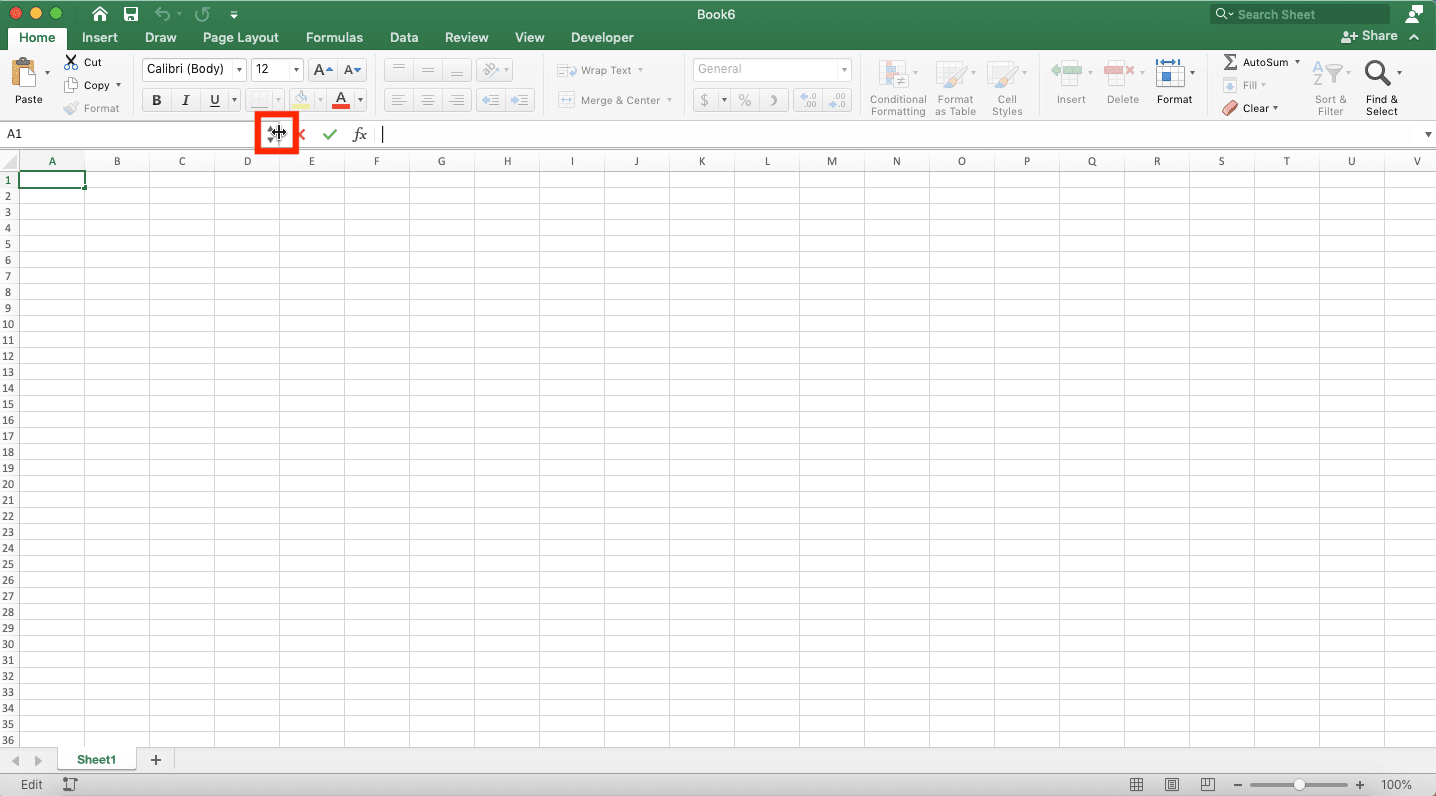Formula Bar in Excel: Function, Definition, and Usage - Compute Expert

# Formula Bar in Excel: Function, Definition, and Usage

Home >> Excel Tutorials from Compute Expert >> Excel Tips and Trick >> Formula Bar in Excel: Function, Definition, and Usage

In this tutorial, you will learn completely about the formula bar feature in excel. Formula bar can help your data input and processing process in an excel file.

Disclaimer: This post may contain affiliate links from which we earn commission from qualifying purchases/actions at no additional cost for you. Learn more

## Formula Bar Definition

The formula bar is a long box on the top of your excel file that contains the cell’s input currently highlighted. This input can be in the form of data or a formula.

## Formula Bar Location

Your formula bar is placed in the top right of your excel file. To make it clearer, take a look at the red boxed part of the following screenshot.## Formula Bar Functions

Generally, there are three formula bar functions in excel. The formula bar:
• Helps to see and edit a formula in a cell, especially if the formula is very long
• Activates the excel formula builder feature more practically
• Accepts or reject the last edit process result on a cell

The last two functions can be done by using the buttons on the left of the formula bar. A more detailed explanation about these buttons will be given in the next part of this tutorial.

## Formula Bar Buttons: Enter, Cancel, dan Insert Function

The formula bar’s helper buttons location can be seen in the following screenshot.Explanation about these three buttons and their functions is as follows.
• Enter: The most left button with the checkmark symbol (V). Can be used to accept a cell edit result after we finish doing the editing process
• Cancel: The middle button with the cross symbol (X). Can be used to reject an edit result and return the cell input state before the editing process is done
• Insert Function: the most right button with the fx symbol. Can be used to activate the formula bar feature in excel. The formula builder is used to input a formula in your cell with the help of some instructions from excel

## How to Show/Hide the Formula Bar

To show/hide the formula bar, add/remove a checkmark in the formula bar checkbox in the View tab. The checkbox location can be seen in the screenshot below.You can add/remove the checkmark by clicking the checkbox. Add the checkmark to show your formula bar and remove the checkmark to hide it.## How to Expand/Collapse the Formula Bar

The formula you input in your cell is very long so your formula bar isn’t enough to hold it? Or, quite the opposite, you want to reduce your formula bar size because it distracts your sheet interface? You can do both of them by expanding/collapsing your formula bar’s height/width.

The way to do it is quite easy. Just move your cursor to the formula bar’s bottom line if you want to change its height. Or if you want to change its width, move your cursor to the left line of your formula bar. Move the cursor until its shape is changed like the ones in the below’s screenshots.

Change the height:Change the width:After your cursor is shaped like that, click and drag it to the direction of the height/width you prefer. Release the drag when the formula bar’s size already fits and the resizing of your formula bar is done!

## Formula Bar Shortcut

Want to expand/collapse your formula bar’s height without having to drag? Excel also provides a shortcut for that purpose.

Just press the Ctrl + Shift + U (Control + Shift + U) buttons simultaneously and your formula bar is automatically expanded/collapsed!

Of course, you can edit a cell content that isn’t a formula in the formula bar. However, this is seldomly done because it is more practical to edit it directly in your cell (enter its edit mode by double-clicking the cell which data you want to edit).

Related tutorials for you to learn:

Get updated excel info from Compute Expert by registering your email. It's free!